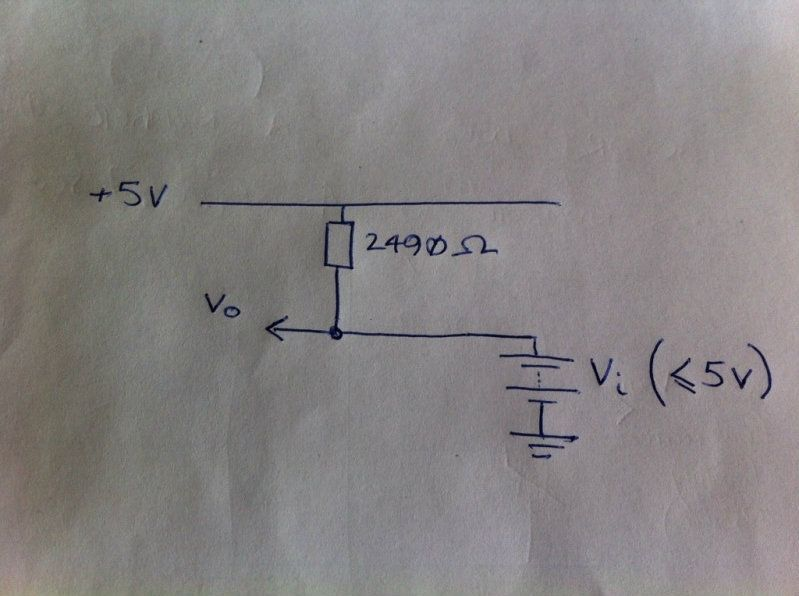# Confused as to what will happen with multiple voltages

Here is (I think) the circuit that forms part of my project:The value of the resistor (2490 Ohms) is fixed. Vi is a variable voltage (driven by the Arduino PWM output - might be filtered but let's just assume it's a nice, clean DC voltage which will never be greater than 5V). What will Vo be or is it not possible to tell? If it were possible to disconnect the resistor, then I'd do it, but it's inside some other electronics to which I don't have access (a Megasquirt EFI controller).

Also tempted to put a diode in between the Arduino (Vo) output and the node but not sure if this is necessary?

Vo will also be exactly 0 or 5 volts since the arduino output will sink the tiny current flowing through the resistor when it's LOW (0 volts)

The value of Vo depends on the source resistance of the voltage source Vi. If we call this resistance R, then in the absence of a load:

Vo = ((5 * R) + (Vi * 2490))/(2490 + R)

So it all depends on the value of R. If the voltage source is an Arduino pin followed by a simple RC network to smooth out the PWM, then R is the resistor used in the RC network plus about 25 ohms.

Looking at it simply: On the basis that Vo is not required to supply current, the resistor is irrelevant to your calculations. Since Vo is electrically tied to Vi then Vo is always equal to Vi.

This, of course assumes the output impedance of Vi is zero. In reality, if Vi output port has impedance, let us call it Vi(imp), when set low, then the value at Vo will be the algebraic solution of the potential divider set up by your 2490 resistor in series with Vi(imp) and the actual value of Vi(low).

Similarly the value of Vo, with Vi set high, will be dependant upon the actual value of Vi(high) and the impedance of its output when set high.

What will Vo be

Vo = Vi.

Mr Henry,

You are perfectly correct.

My bullsh*t statement is exactly that ; since Vi is the voltage seen at the output terminals and is directly connected to Vo, then Vo = Vi. The impedance of the output port and the respective Vi(low) and Vi(high) are of no consequence.

Happy new year

If Vo = Vi then that's great. Thanks for all the replies - I think I'll breadboard it and hook up a DVM to check!

jackrae: I hear you. It is always easy to complicate matters.

If Vo = Vi then that's great.

Look at it this way: Vi and Vo are connected via a wire (of zero resistance). So Vi cannot differ from Vo.

Now, if Vi is the specified voltage on that battery with high output resistance, it gets slightly more interesting. But a regular battery / power supply will have output impedance far lower than that resistor's (2490ohm) so even if they differ, it is immaterial.

I hope you are right (and that my simplified circuit is a sufficient analog of the real intended circuit!

spandit: I hope you are right (and that my simplified circuit is a sufficient analog of the real intended circuit!

You haven't said what your real intended circuit is, so we don't know whether that is true or not.

dc42:

spandit: I hope you are right (and that my simplified circuit is a sufficient analog of the real intended circuit!

You haven't said what your real intended circuit is, so we don't know whether that is true or not.

Fair point! The node (where we're measuring Vo) is the input to a Megasquirt EFI controller which normally measures the voltage across a potential divider (between the 2490Ohm resistor and a thermistor). I'm giving it a voltage feed from an Arduino instead (the software is taking the average of 4 thermistors and combining them into 1 output whilst also displaying all 4 on an LCD bargraph). It's more or less finished but probably needs a few tweaks

spandit: Fair point! The node (where we're measuring Vo) is the input to a Megasquirt EFI controller which normally measures the voltage across a potential divider (between the 2490Ohm resistor and a thermistor). I'm giving it a voltage feed from an Arduino instead (the software is taking the average of 4 thermistors and combining them into 1 output whilst also displaying all 4 on an LCD bargraph). It's more or less finished but probably needs a few tweaks

And how are you deriving a voltage feed from the Arduino? If it's from a PWM pin and simple RC smoothing filter, then you need to take the source resistance of the RC filter into account. If you follow the RC filter with an op amp used as a unity-gain buffer, then its output resistance will be low enough to ignore.